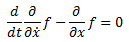Derivation of the Euler-Lagrange Equation

We can also use those tools to develop the Euler-Lagrange equation itself.  In my humble opinion, this is a more straight forward derivation than is usually shown.

If L = T – V, with T a particle’s kinetic energy and V its potential energy, L is called the Lagrangian and the integral of the Lagrangian over a path in time is called the action.  The principle of least action says that the path a particle actually takes is a minimum in action.

For a simple example, the Lagrangian of a particle in a uniform gravitational field isJumping to the answer, the Euler-Lagrange equation isPlugging in, we eventually getWhich of course is obvious, from Newton’s second law.

Now let’s derive the equation on our own.

We have a function f that depends on x coordinate, x’s derivative in time x-dot, and t time.  Time varies between t=a and t=b.  We want to minimize the integral:We can make this a discrete Riemann sum by dividing time up into even time slices, t = a, t = a + delta, t = a + 2 delta, etc.

The first term isThe second term isThe second x value is determined by x-dot1 and delta, but there is no way of determining x-dot2 from anything else (yet).  So the x-dots just have to be the independent variables.

Altogether, the integral isNote that each x-dotq appears once in the x-dot position, and appears in each x position for each i greater than q.  So when we take the gradient, we getNow we divide by delta and think about this equation.  The first term is simply the partial derivative of f with respect to x-dot at some arbitrary time t.  The second term is the integral (because it is a Riemann sum) of (the partial derivative of f with respect to x) from that time t to b.  In other words (or symbols)Now differentiate that with respect to t and we getwhich is the equation we wanted to derive.

2 thoughts on “Derivation of the Euler-Lagrange Equation”

1.admin on said:

Correction: Since the equation was divided by delta, the second to last equation is not equal to the gradient of S.

Also, you might argue I’m being lax in my use of a dummy variable in that equation. If the lower limit was t0, and we differentiated with respect to that, the final result might be slightly more obviously true.

2.admin on said:

Also note that this derivation doesn’t require you to “magically” know the final state before deriving it.